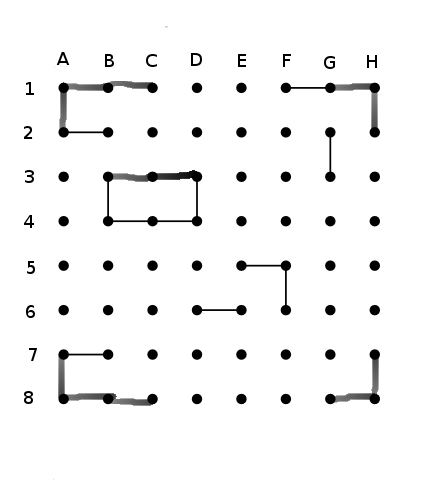Rules of Round Trip

## Rules of Round Trip

The Round Trip puzzle has all the qualities of a good puzzle: simple and challenging. Supposedly this puzzle was invented in the year 1990 and this puzzle is relatively unknown now, I will be happy if it become mote popular because the Round Trip is very pleasant to solve.

The goal is to draw a loop in the grid visiting each dot only once. At the begining some lines are given, your task is to draw more lines in accordance with the rule: Forming a closed loop visiting each dot.

This is a puzzle with the solution, notice that the loop visits each point.Then you must ceate a path visiting each dot by adding lines (vertical or horizontal - never diagonaly) so that at the end the path form a closed loop. Each puzzle is solvable by logic. This puzzle could have different size from 4x4 to 18x18.

## SOME TIPS

Let try to solve a 8X8 puzzle (figure 1) to illustrate some tips, each colomn and row is labeled by letter and number to ease the explanations.

The first thing to remember is that each dot t is visited only once, so this means that only two lines must link the dot: the entry line and the exit line. We could concude an important fact with this information: if a dot has only two possible ways to be linked then these ways must be drawn. So at the begining of each puzzle this situation appears because the dots situated at each corner have only two possible ways. So this is a free 8 lines !!!, just at starting the puzzle  (see figure 2).

Now I will illustrate a situation that you must avoid, look at the dot B1, it has already an input line coming from A1, only two other ways are possible for the other line: a line going to C1 or going to B2. The problem is if the line goes to B2 (see figure 3) it will create a closed loop amoung the four dot in the corner (A1,B1,A2 and B2) and it will be impossible to create one single loop linking all the dots.

So for the dot B1 the way to B2 is eliminated then the only possible way left is a line going to C1 (figure 4). By the same reasonnnig we add a line from B8 to C8.

.

The following tip is helpful because you could use it often. Look at the dot C3, it has must have two lines for a possibilty of three directions: C2,B3 and D3 ( the dot C4 is eliminated because this dot has already two lines). But one possibe configuration is that C3 has a line to B3 and D3 (figure 5).Figure 5

These two lines cause a forbiden situation ( a closed loop not linking all the dots), so it means that at least a line must go to C2, even if we dont know where the other line goes (B3 or D3), we could draw the line from C3 to C2 (figure 6).

Now, two more dots have only two possible ways (A3 and A6). We are able to draw the only two possible lines for each. And it result that the dots A4 and A5 have only one possible way to have a line (figure 7).